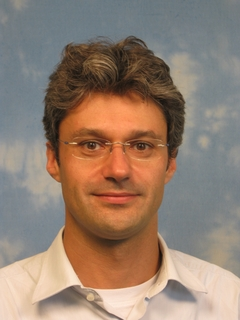# Dynamical low rank approximation of random time dependent PDEs#### Keywords

Advanced Numerical Methods for Scientific Computing
Speaker:
Fabio Nobile
Affiliation:
Mathematics Institute, CSQI, Ecole Polytechnique Fédérale de Lausanne, Switzerland
When:
Thursday 29th June 2017
Time:
14:00:00
Where:
Aula Consiglio, VII piano - Dipartimento di Matematica, Politecnico di Milano - Edificio 14
Abstract:
Partial differential equations with random coefficients and input data (random PDEs in short) arise in many applications in which the data of the PDE need to be described in terms of random variables/fields due either to a lack of knowledge of the system or to its inherent variability. The numerical approximation of statistics of the solution poses several challenges when the number of random parameters is large and/or the parameter-to-solution map is complex, and effective surrogate or reduced models are of great need in this context. In this talk we consider time dependent PDEs with few random parameters and seek for an approximate solution in separable form that can be written at each time instant as a linear combination of linearly independent spatial functions multiplied by linearly independent random variables (low rank approximation) in the spirit of a truncated Karhunen-Loève expansion. Since the optimal deterministic and stochastic modes can significantly change over time, we consider here a dynamical approach where those modes are computed on the fly as solutions of suitable evolution equations. From a geometrical point of view, this corresponds to constraining the original dynamics to the manifold of fixed rank functions, i.e. functions that can be written in separable form with a fixed number of terms. Equivalently, the original equations are projected onto the tangent space to the manifold of fixed rank functions along the approximate trajectory, similarly to the Dirac-Frenkel variational principle in quantum mechanics. We discuss the construction of the method as well as practical numerical aspects for several time dependent PDEs with random parameters, including the heat equation with a random diffusion coefficient; the incompressible Navier-Stokes equations with random Dirichlet boundary conditions; the wave equation with random wave speed. In the latter case, we propose a dynamical low rank approximation that preserves the symplectic structure of the equations.
Note:
Fabio Nobile is associate professor in Mathematics at the Ecole Polytechique Federale de Lausanne, Switzerland, since 2011. Previously he has been assistant professor in numerical analysis at Politecnico di Milano. He graduated in electronic engineering at Politecnico di Milano in 1998; PhD in Applied Mathematics at EPFL in 2001; 2 year post-doc position (ICES fellowship) at ICES, University of Texas at Austin. Invited speaker to several international conferences and workshops, including the 7th European Congress of Mathematics 7ECM in 2016. Co-Chair and main organizer of the SIAM UQ16 conference, EPFL. Awarded the second prize at the 12th Leslie Fox Prize in 2005. He is author of more than 60 publications on international journals. He is member of the editorial board of 5 international journals. His main research activity is in numerical approximation of PDEs with stochastic coefficients, sparse approximation techniques, numerical solution of fluid-structure interaction problems, simulation of the cardiovascular system.